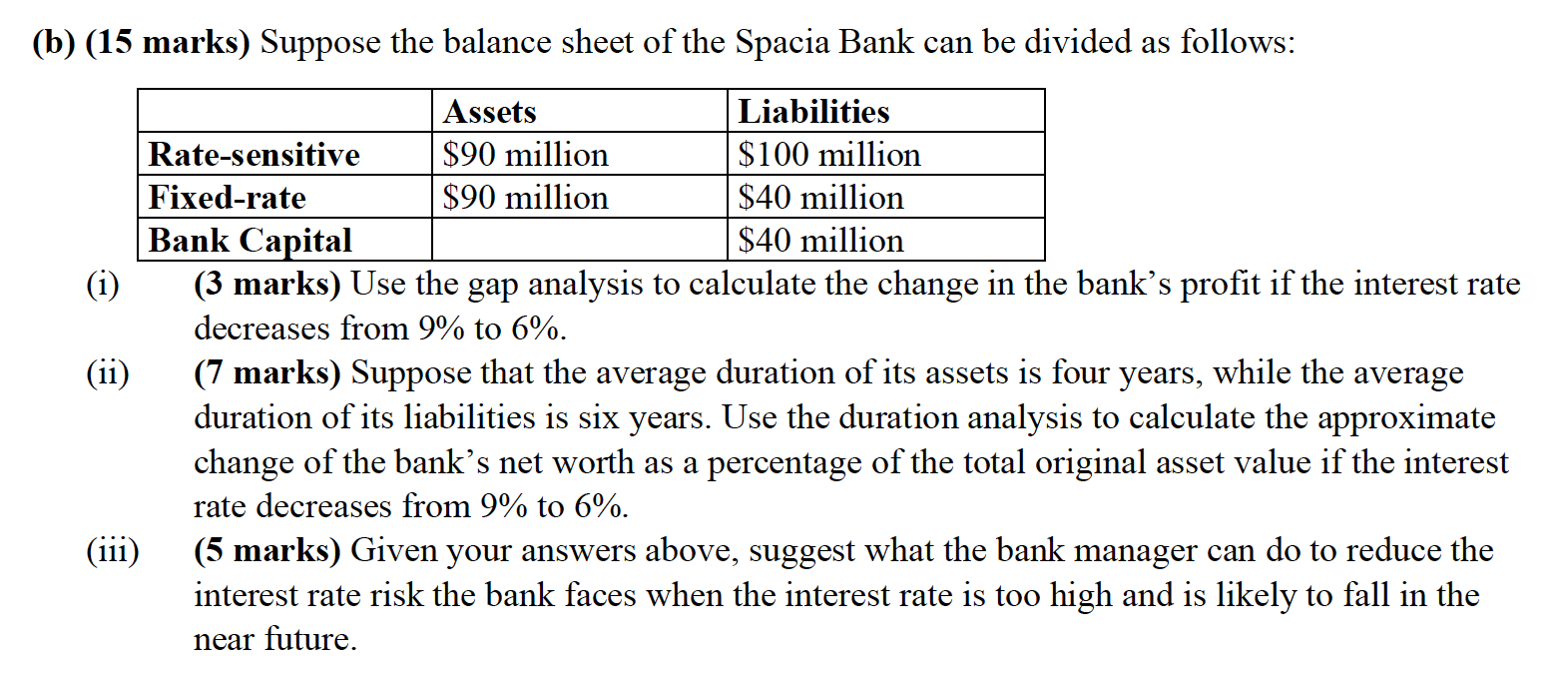# Question (b) (15 marks) Suppose the balance sheet of the Spacia Bank can be divided as follows: Assets Liabilities Rate-sensitive \$90 million \$100 million Fixed-rate \$90 million \$40 million Bank Capital \$40 million (i) (3 marks) Use the gap analysis to calculate the change in the bank's profit if the interest rate decreases from 9% to 6%. (ii) (7 marks) Suppose that the average duration of its assets is four years, while the average duration of its liabilities is six years. Use the duration analysis to calculate the approximate change of the bank's net worth as a percentage of the total original asset value if the interest rate decreases from 9% to 6%. (iii) (5 marks) Given your answers above, suggest what the bank manager can do to reduce the interest rate risk the bank faces when the interest rate is too high and is likely to fall in the near future.